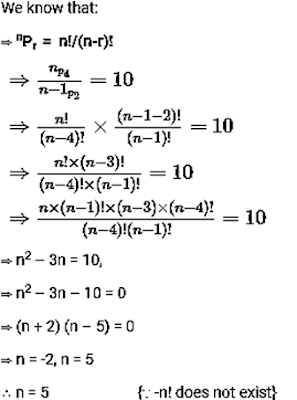## Permutations and Combinations Practice Questions

Permutations and Combinations Practice Questions
These Permutation and Combination questions/problems with solutions provide you vital practice for the topic. The purpose of these posts is very simple: to help you learn through practice. Solve these problems to succeed in upcoming SBI Clerk/PO exams 2018.

1. In how many different ways can 2 vowels and 3 consonants be selected from 4 vowels and 10 consonants?
a) 720
b) 840
c) 620
d) 240
e) None of these
2. Find the number of ways in which 6 players out of 11 players can be selected so as to include 3 particular players.
a) 56
b) 54
c) 45
d) 65
e) None of these
3. In how many ways can 3 people be seated in a row containing 6 seats?
a) 110
b) 130
c) 140
d) 120
e) None of these
4. How many 4 letter code can be formed using the first 9 letters of the English alphabets, if no letter can be repeated?
a) 3024
b) 3036
c) 3021
d) 3034
e) None of these
5. In how many different ways can the letters of the word ‘ASSASINATION’ be arranged?
a) 1221846
b) 3234464
c) 3326400
d) 3126408
e) None of these
6. If  np4 = 10 × n-1p2, find the value of n = ?
a) 5
b) 4
c) 10
d) 12
e) None of these
7. Out of 5 men and 3 women, a committee of 3 persons is to be formed. In how many ways can it be formed selecting at least 2 women?
a) 15
b) 16
c) 21
d) 56
e) None of these
8. There are 35 teachers in a school. In how many different ways one principal and one vice principal can be chosen?
a) 1190
b) 1160
c) 1170
d) 1180
e) 1090
9. For how many integers between 10 and 100 in the tens digit equal to 5, 6 or 7 and the units digit (ones digit) equal to 2, 3, 4 ?
a) three
b) four
c) six
d) nine
e) None of these
10. Four boys and three girls are to be seated for a dinner such that no two girls sit together and no two boys sit together. Find the number of ways in which this can be arranged.
a) 144
b) 36
c) 72
d) 180
e) None of these

Solutions:
1. A) Two vowels can be selected from 4 in 4C2 ways. Three consonants can be selected from 10 in 10C3 ways.
2 vowels and 3 consonants can be selected in (4C2 × 10C3) ways
= (4 * 3)/(2 * 1) × (10 * 9 * 8)/(3 * 2 * 1) = 720
2. A) Since, 3 particular players are always included, the choice now reduces to only 3 players among 8 players which can be done in 8C3 ways.
8C3 = 8!/(3! 5!) = (8 * 7 * 6 * 5!)/(3 * 2 * 1 * 5!) = 8 * 7 = 56
3. D) First person can be seated in 6 ways, the second person in 5 ways and the third person in 4 ways.
Then, by fundamental principle, total number of ways in which three persons can be seated in 6 seats in a row is (6 × 5 × 4) ways = 120 ways
4. A) By fundamental principle, it is (9 × 8 × 7 × 6) ways = 3024 ways
5. C)ASSASINATION’ contains 12 letters which can be grouped as follows.
A = 3, S = 3, I = 2, N = 2, T = 1, O = 1
Required permutations = 12!/(3! * 3! * 2!* 2!) = 3326400
6. A)7. B) Case – I
Committee contains 2 women and 1 men.
No. of ways of forming this committee = (number of ways of choosing 2 women out of 3) × (number of ways of choosing 1 man out of 5)
3C2  5C1 =3 × 5 = 15
Case – II
Committee contain 3 women and 0 men
No. of ways of forming this committee = number of ways of choosing 3 women out of 3.
3C=1
Total number of ways = 15 + 1 = 16
8. A) Ways of choosing a principal from 35 teacher = 35.
Now, after choosing principal, no. of ways of choosing Vice principal = 34 [ 35 1 = 34]
Hence, no of ways for selecting a principal and a vice – principal
= 35 × 34
= 1190 way
9. D) For the tens place we have 3 choices.
And for the units place we have 3 choices.
Total number of integers we can form = 3 × 3 = 9
10. A) Since no two girls and no two boys sit together it means they sit on the alternate positions.
Therefore first of all we arrange 3 girls in 3! ways then we arrange 4 boys in newly created 4 places in 4P4 ways
Thus the total number of arrangements = 3! × 4! = 144